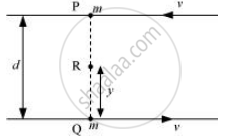CBSE (Science) Class 11CBSE
Share

Two Particles, Each of Mass M And Speed V, Travel in Opposite Directions Along Parallel Lines Separated by a Distance D. Show that the Vector Angular Momentum of the Two Particle System is the Same Whatever Be the Point About Which the Angular Momentum is Taken - CBSE (Science) Class 11 - Physics

ConceptTorque and Angular Momentum

Question

Two particles, each of mass and speed v, travel in opposite directions along parallel lines separated by a distance d. Show that the vector angular momentum of the two particle system is the same whatever be the point about which the angular momentum is taken.

Solution

Let at a certain instant two particles be at points P and Q, as shown in the following figure.Angular momentum of the system about point P:

vecL_p = mv xx 0 + mv xx d

= mvd ....(i)

Angular momentum of the system about point Q:

vecL_Q = mv xx d + mv xx 0

= mvd   ....(ii)

Consider a point R, which is at a distance y from point Q, i.e.,

QR = y

∴PR = d – y

Angular momentum of the system about point R:

vecL_R = mvxx(d-y) + mv xx y

= mvd - mvy + mvy

=mvd ...(iii)

Comparing equation i, ii and iii we get

vecL_p = vecL_Q = vecL_R .... (iv)

We infer from equation (iv) that the angular momentum of a system does not depend on the point about which it is taken

Is there an error in this question or solution?

APPEARS IN

NCERT Solution for Physics Textbook for Class 11 (2018 to Current)
Chapter 7: System of Particles and Rotational Motion
Q: 7 | Page no. 178

Video TutorialsVIEW ALL 

Solution Two Particles, Each of Mass M And Speed V, Travel in Opposite Directions Along Parallel Lines Separated by a Distance D. Show that the Vector Angular Momentum of the Two Particle System is the Same Whatever Be the Point About Which the Angular Momentum is Taken Concept: Torque and Angular Momentum.
S